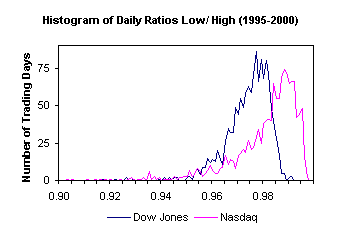# AnalyticBridge

A Data Science Central Community

The following theorems are related to the stock market and trading strategies. They have roots in the martingale theory, random walk processes, gaming theory or neural networks. We present some of the most amazing and deep mathematical results, of practical interest to the curious trader. If you think of a result worth publishing here, please submit it via E-mail. We will acknowledge your contribution.

Lorenz Curve

Let's say that one makes 90% of his trading gains with 5% of his successful trades. We writeh(0.05) = 0.90. The function h is known as the Lorenz curve. If the gains are the realizations of a random variable X with cdf F and expectation E[X], then

h(x) = ( ∫[0,x] F -1(v) dv ) / E[X],       0 ≤ x ≤ 1.

To avoid concentrating too much gain on just a few trades, one should avoid strategies that have a sharp Lorenz curve. The same concept applies to losses. Related keywords: inventory management, Six Sigma, Gini index, Pareto distribution, extreme value theory.

(posted by Vincent Granville, from Data Shaping Solutions)

Black-Scholes Option Pricing Theory

The Black-Scholes formula relates the price of an option to five inputs: time to expiration, strike price, value of the underlier, implied volatility of the underlier, the risk-free interest rate. For technical details, check out www.hoadley.net. You may also look at the book A Probability Path by Sidney Resnik (Ed. Birkhauser, 1998).

The formula can be derived from the theory of Brownian motions. It relies on the fact that stock market prices can be modeled by a geometric Brownian process. The model assumes that the variance of the process does not change over time, and that the drift is a linear function of the time. However these two assumptions can be invalidated in practice.

(posted by Martin Sewell, from University College London)

Discriminant Analysis

Stock picking strategies can be optimized using discriminant analysis. Based on many years of historical stock prices, it is possible to classify all stocks in three categories - bad, neutral or good - at any given time. The classification rule must be associated with a specific trading strategy, such as buying at close today and selling at close seven days later. Data Shaping Solutions is currently investigating this approach.

Generalized Extreme Value Theory

What is the parametric distribution of the daily ratio high/low? Or the 52-week high/low? And how would you estimate the parameter for a particular stock? Interdependencies in the time series of stock prices make it difficult to compute an exact theoretical distribution.The distribution is characterized by two parameters: mode and interquartile. The Nasdaq has a much heavier lefthand tail, making it more attractive to day traders. As a rule of thumb, stocks with an heavy lefthand tail are good picks for Data Shaping strategies.

(posted by Jean-Paul Rasson, from University of Namur)

Random Walks and Wald's identity

 Let us consider a random walk in Z, with transition probabilities P(k to k+1)=p, P(k to k)=q, P(k to k-1)=r, with p+q+r=1. The expected number of steps for moving above any given starting point is infinite if p is smaller than r. It is equal to 1/(p-r) otherwise.

This result, applied to the stock market, means that under stationarity conditions (p=r), investing in a stock using the buy and hold strategy may never pay off, even after an extremely long period of time.

(posted by Vincent Granville, from Data Shaping Solutions

Arcsine Law

This result explains why 50% of the people consistently lose money, while 50% consistently win. Let's compare stock trading to coin flipping (tails = loss, heads = gain). Then

• The probability that the number of heads exceeds the number of tails in a sequence of coin-flips by some amount can be estimated with the Central Limit Theorem and the probability gets close to 1 as the number of tosses grows large.
• The law of long leads, more properly known as the arcsine law, says that in a coin-tossing games, a surprisingly large fraction of sample paths leave one player in the lead almost all the time, and in very few cases will the lead change sides and fluctuate in the manner that is naively expected of a well-behaved coin.
• Interpreted geometrically in terms of random walks, the path crosses the x-axis rarely, and with increasing duration of the walk, the frequency of crossings decreases, and the lengths of the “waves” on one side of the axis increase in length.

(posted by Vincent Granville, from Data Shaping Solutions

Views: 5907

### Replies to This Discussion

Could you explain better the x-axis of the plot in the "Generalized Extreme Value Theory" section? Is it the
high/low ratio? If so it cannot be by definition less than 1?